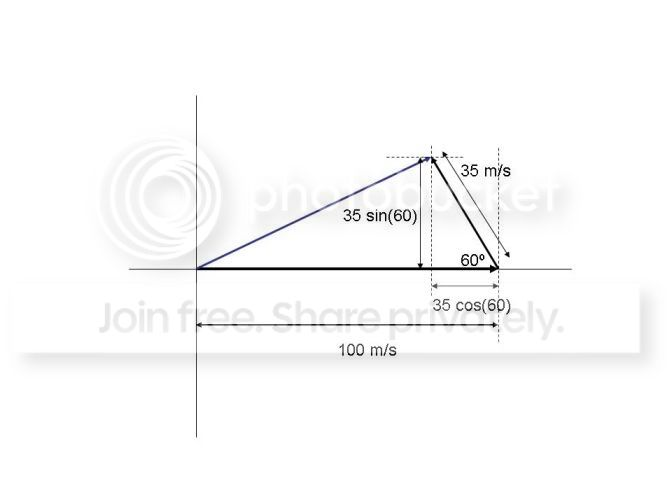User Name Remember Me? Password

 Kinematics and Dynamics Kinematics and Dynamics Physics Help ForumSep 7th 2010, 01:44 PM #1 Junior Member   Join Date: Sep 2010 Posts: 1Simple reference frame problem You are on an airplane traveling due east at 100 m/s with respect to the air. The air is moving with a speed 35 m/s with respect to the ground at an angle of 30° west of due north. What is the speed of the plane with respect to the ground? __________ So here's what Im doing: Drew the whole right triangle thing to bring down air into its horizontal and vertical components. Vertical turned out to be 17.5m/s and horizontal 30.31m/s. Then I broke up the speed of the plane into its horizontal and vertical components, but I used the same angle of 30 to get horizontal speed of 86.6 and vertical speed of 50. I'm pretty sure this part is wrong. So to get the speed of the plane wrt ground, the equation is: V[p,g]=V[p,a] + V[a,g] so v[p,g]=86.6+30.31=116.91. That was the wrong answer. I tried subtracting-wrong answer. I even used 60 degrees instead of 30 for breaking up the plane into vertical and horizontal, but that didnt work out either. I have no idea what Im doing.Sep 8th 2010, 10:16 AM #2 Physics Team   Join Date: Jun 2010 Location: Morristown, NJ USA Posts: 2,347 You're on the right track with respect to breaking the components of velocity into horizontal and vertical pieces. It helps to draw out a sketch - see below. First, you gave the angle of the wind as 30 degrees west of north, which means it is 60 degrees from teh horizontal axis. The horizontal component of the wind is therefore -35m/s x cos(60) = -17.5 m/s, and the vertical component is 35 m/s x sin(60) = 30.31 m/s. So that was the first problem - you had these reversed. Note the minus sign for the horizontal piece - that's because it is the west (left). The horizontal component of the wind is +100 m/s, and the vertical component is 0 m/s - since the plane is heading due east. Now add the horoizontal pieces and then the vertical pieces: V_h = 100 - 17.5 = 82.5m/s V-v = 30.31 m/s The total velocity is found using the Pythagorian theorem: V_t = sqrt (V_h^2 + V_v^2) = sqty (82.5^2 + 30.31^2) = 87.9 m/s Hope this helps.Tags frame, problem, reference, simpleThread ToolsShow Printable VersionEmail this Page Display ModesLinear ModeSwitch to Hybrid ModeSwitch to Threaded ModeSimilar Physics Forum Discussions Thread Thread Starter Forum Replies Last Post PhysicsKidd Kinematics and Dynamics 3 Nov 5th 2014 01:13 PM avengefulghost Kinematics and Dynamics 1 Jan 16th 2011 07:56 PM HassanZahid Special and General Relativity 1 Dec 24th 2008 04:35 PM chicomore Kinematics and Dynamics 0 Dec 5th 2008 05:41 PM evabern Special and General Relativity 1 Oct 15th 2008 10:58 AM